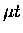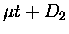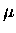Next: MMC ro-vibrational relaxation Up: Back-decayed energy distribution Previous: Back-decayed energy distribution

## MMC recoil and thermalization

In this section, we shall take a more detailed look at each step in the back decay in order to estimate the energy ofafter back decay.

When the muonic molecular complex is formed in the collision, it will receive recoil velocity . For the case ofcollisions at the main resonance of eV, cms-1. The final velocity of MMC is important since it affects the lab energy of back-decayed. Two kinematic extremes are the complete thermalization of MMC before it decays, and no thermalization at all. For either case, the maximum possible energy of back-decayed(for a low temperature target) is obtained when elastic scattering'' (i.e. no excitation of the target D2 molecule) takes place. However, even in elastic scattering,is decelerated in the lab frame due to the recoil of MMC and D2 as discussed above.

Cross sections for the interaction of has been calculated by Padial et al. [154,239]. Extrapolating a figure given in Ref.  to epithermal energies, the elastic scattering cross section appears to have a value of order cm-2. The average time between elastic collision , where n is the D2 number density, can be compared to the MMC life time, .

In the case of eV, which corresponds to the largest resonance for molecular formation in, the MMC recoil velocity is cms-1, hence at a molecular density of 1.4 N0/2 ( cm-3, the atomic density of liquid hydrogen) corresponding to a solid at 3 K, s. Comparing this to  [156,152], we have

 (133)

suggesting a fair number of collisions occur before the MMC decays.

The average number of collisions needed to slow the MMC of EMMCinitto EMMCfin, can be roughly estimated, by generalizing the formula for neutron thermalization :

 (134)

where

 (135)

For eV in, the MMC recoil energy is eV, and we have for EMMCfin=0.4 meV. Obviously, the formula B.10 gives simply a crude estimate, but together with Eq. B.9, one can assume that the MMC slows down significantly before back decay occurs.Next: MMC ro-vibrational relaxation Up: Back-decayed energy distribution Previous: Back-decayed energy distribution